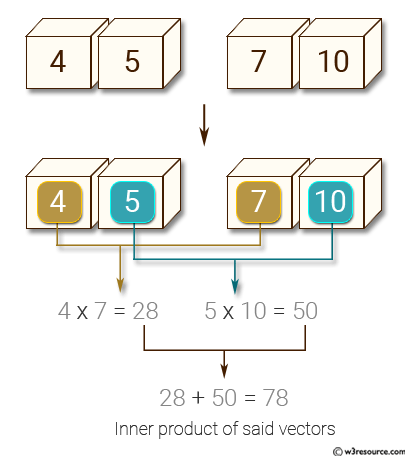﻿ NumPy: Compute the inner product of two given vectors - w3resource# NumPy: Compute the inner product of two given vectors

## NumPy: Basic Exercise-33 with Solution

Write a NumPy program to compute the inner product of two given vectors.

Sample Solution :

Python Code :

``````import numpy as np
x = np.array([4, 5])
y = np.array([7, 10])
print("Original vectors:")
print(x)
print(y)
print("Inner product of said vectors:")
print(np.dot(x, y))
``````

Sample Output:

```Original vectors:
[4 5]
[ 7 10]
Inner product of said vectors:
78
```

Pictorial Presentation:Python Code Editor:

Have another way to solve this solution? Contribute your code (and comments) through Disqus.

What is the difficulty level of this exercise?

Test your Python skills with w3resource's quiz

﻿

## Python: Tips of the Day

Checks if the given number falls within the given range.

Example:

```def tips_range(n, start, end = 0):
return start <= n <= end if end >= start else end <= n <= start
print(tips_range(2, 4, 6))
print(tips_range(4, 8))
print(tips_range(1, 3, 5))
print(tips_range(1, 3))
```

Output:

```False
True
False
True
```